[Documentation] [TitleIndex] [WordIndex]

## ODE Solvers

Please see the ODE's users manual for general ODE documentation.

In general, rigid body simulators solve

• Kinematics constraints
• Collision and contact constraints
• Rigid body dynamics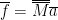ODE's constraint solver uses a full coordinate system approach and enforces joint and contact constraints as posed by the linear complementarity problem (LCP).

### Basic Governing Equations of Constrained Dynamics

Before we discuss the solvers, here is a very brief note here on the governing dynamics equations. Simple Euler's discretization yields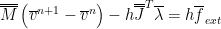Constraints are described by the constraint Jacobian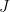given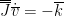or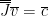where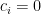for fixed joints and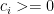for contact joints.

If we rewrite in matrix form we have: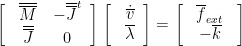Substitute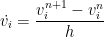and rearrange to get: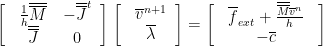Left multiply top row of the matrix equation by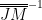, then eliminate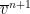from the top row using the equality in the second row (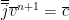) and arrive at: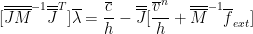ODE is semi-implicit in that the Jacobiansand external forces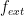from the previous time step are used throughout the iterations.

### Solvers

ODE ships with two default solvers

• Dantzig's Agorithm dWorldStep()

• This algorithm will attempt to achieve a numerically exact solution. It is about one order of magnitude slower than SOR PGS LCP solver and its convergence behavior is less predictable in practice.
• Successive Over-Relaxation (SOR) Projected Gauss-Seidel (PGS) LCP solver dWorldQuickStep()

• Essentially a Gauss-Seidel algorithm with solution vector projected into the allowable solution space at every update. The PR2 robot simulations default to this algorithm running at 1kHz (to match mechanism controller update rate of the real robot).

#### Dantzig's Agorithm

Please refer to step.cpp for implementation details. Various references contain discussions on this algorithm, see 2.7.1 in Michael Cline, "Rigid Body Simulation with Contacts and Constraints" for example. See also the Cottle and Dantzig book for details, Baraff extended the Dantzig algorithm to include friction in his SIGGRAPH 1994 paper. Also, chapter 14 of Murilo Coutinho's book "Guide to Dynamic Simulations of Rigid Bodies and Particle Systems" has detailed introduction to both Dantzig's algorithm and Baraff's friction extention.

The Dantzig algorithm solves general BLCP (Linear Complementarity Problem with Bounds), which has the form:

Solve: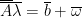such that: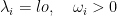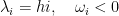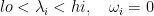In ODE's step.cpp,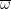is set to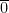, then it has consistent form with SOR PGS LCP: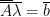The Dantzig algorithm applies to more general BLCP. It incrementally computes intermediate solutions for each entry in the unknown vector: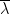. It compute the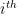unknown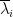without violating the non-interpenetration or box friction conditions for the previousrows that already resolved. Suppose the length ofis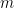, the solution should be obtained after we solve the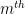unknown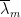.

We first define the different sets based on properties of unknowns: Clamped Set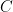is a set of index, with size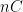that satisfies:Similarly, Non-Clamped Set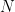is a set of indexthat satisfies:orDo-not-care Set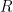is a set of indexthat satisfies: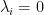where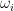could be any value. The permuted index is in the order of: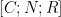.

During execution of Dantzig's algorithm, the left top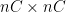clamped matrix of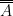, we denote as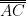, always maintains with an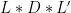(LDLT) factorization.

Procedures of Dantzig's algorithm are: If we have only bounded constraints (bilateral constraints with lower and upper bounds), then all the indices are mapped to set, we do an LDLT factorization of matrix, then solve the LDLT system, we are done.

Else if we have a mixture of unbounded and unbounded constraints, Dantzig algorithm does LDLT factorization and solve the firstunknowns.

When we hit the first friction constraint, compute the corresponding lower and upper bound, using normal force at the same contact.

Assume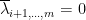, updateand make sure to push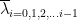to one of the sets:, i.e. don't violate the first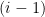constraints, since update onmight break the firstconstraint satisfaction.

Once we finish a complete loop on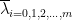, the solution is found.

#### SOR PGS LCP

As implemented in ODE's quickstep.cpp, and reiterating the solution procedure from several popular literatures here.

We are essentially solving a system of linear equations where the solution space is non-negative in parts of the system.where based on the derivations from governing equations in the previous section,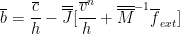and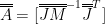If we solve forin delta-form using Gauss-Seidel, i.e.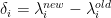then it follows that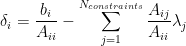for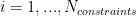Formulate the desired solution in the form of acceleration1 (inverse mass matrix times constraint forces), denoted by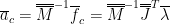thenupdate becomes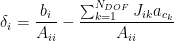and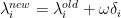for, where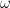is the relaxation parameter.

where each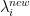is projected into its corresponding solution space depending on the type of constraint specified.

At every iteration, for eachupdate above, constraint accelerations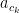are updated in the following manner: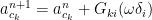for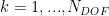where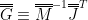For more details please see the list of references.

1. to clarify, in quickstep.cpp, $$\bar{a}_c$$ is denoted by variable fc as of svn revision 1675 (1)

2019-12-07 12:55